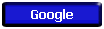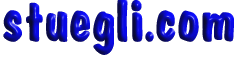# Impulse of Force

The product of average force and the time it is exerted is called the impulse of force. From Newton's second lawthe impulse of force can be extracted and found to be equal to the change in momentum of an object provided the mass is constant:Calculation

The main utility of the concept is in the study of the average impact force during collisions. For collisions, the mass and change in velocity are often readily measured, but the force during the collision is not. If the time of collision can be measured, then the average force of impact can be calculated.

# Minimizing Impact Force

The process of minimizing an impact force can be approached from the definition of the impulse of force:If an impact stops a moving object, then the change in momentum is a fixed quantity, and extending the time of the collision will decrease the impact force by the same factor.

Alternatively, the same scenario can be examined with the aid of the work-energy principle.Car crash example

An impact which stops a moving object must do enough work to take away its kinetic energy, so extending the distance moved during the collision reduces the impact force.

# Airplane and Duck

Estimate the average impact force between an airliner traveling at 600 mi/hr and a 1 pound duck whose length is 1 foot. This is an example of the use of impulse of force.# Airplane & Duck Force Estimate

For the airplane and duck force estimate, the mass of the duck is needed, but the weight in the U. S. Common system of units is given. The mass is# Airplane & Duck Estimates

For the airplane and duck force estimate, the mass of the duck is determined, but the change in velocity and time of collision must be estimated in order to estimate the average impact force.

The change in velocity of the duck is estimated to be 600 mi/hr = 880 ft/s by assuming a head on collision, assuming that the duck is riding with the airliner after the collision, and assuming that the duck's velocity is negligible compared to that of the airliner, the "hovering duck" approximation.The time of collision is assumed to be the time of transit of the duck's dimension of 1 foot, so 1/880 second.

# Airplane & Duck Collision Force

As a variation on the airplane and duck problem, change the mass of the bird and speed of the aircraft. Enter a length of the bird for estimation of the impact time.

Mass of bird m= kg, weight = N = lb.
Velocity of aircraft = m/s = km/h = mi/h, which for bird length = cm gives an estimated impact time of seconds. Under these conditions, the impact force is
Average impact force = N = lb = tons.Copyright © 2005 -  S. B. EglI